# Simultaneous equations

### Term 1 starting in week 10 :: Estimated time: 3 weeks

• Understand that equations can have more than one solution
• Determine whether a given (x, y) is a solution to a pair of linear simultaneous equations
• Solve a pair of linear simultaneous equations by substituting a known variable
• Solve a pair of linear simultaneous equations by substituting an expression
• Solve a pair of linear simultaneous equations using graphs
• Solve a pair of linear simultaneous equations by subtracting equations
• Solve a pair of linear simultaneous equations by adding equations
• Use a given equation to derive related facts (review)
• Solve a pair of linear simultaneous equations by adjusting one equation
• Solve a pair of linear simultaneous equations by adjusting both equations
• Form a pair of linear simultaneous equations from given information
• Form and solve pair of linear simultaneous equations from given information

For higher-attaining pupils:

• Determine whether a given (x, y) is a solution to both a linear and quadratic equation
• Solve a pair of simultaneous equations (one linear, one quadratic) using graphs
• Solve a pair of simultaneous equations (one linear, one quadratic) algebraically
• Solve a pair of simultaneous equations involving a third unknown

This page should remember your ticks from one visit to the next for a period of time. It does this by using Local Storage so the information is saved only on the computer you are working on right now.

## Lesson Starters

Here are some suggestions for whole-class, projectable resources which can be used at the beginnings of each lesson in this block.

### 1st Lesson#### Oblongs

Find the dimensions of a rectangle given the perimeter and area.

### 2nd Lesson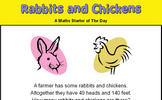#### Rabbits and Chickens

There are some rabbits and chickens in a field. Calculate how many of each given the number of heads and feet.

### 3rd Lesson#### Rail Weigh

Use the weights of the trains to work out the weight of a locomotive and a coach. A real situation which produces simultaneous equations.

### 4th Lesson#### Simultaneous Occasions

A problem which can best be solved as a pair of simultaneous equations.

### 5th Lesson#### Summer Holidays

How many children and how many donkeys are on the beach? You can work it out from the number of heads and the number of feet!

### 6th Lesson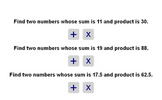#### Two Numbers

Find the two numbers whose sum and product are given.

### 7th Lesson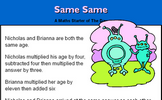#### Same Same

A problem involving two people's ages which can be solved using algebra.

### 8th Lesson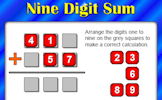#### Planet Numpair

The sum and product are given, can you find the two numbers?

### 9th Lesson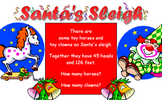#### Santa's Sleigh

Work out the number of clowns and horses given the number of heads and feet.

Some of the Starters above are to reinforce concepts learnt, others are to introduce new ideas while others are on unrelated topics designed for retrieval practice or and opportunity to develop problem-solving skills.

White Rose ResourcesEnd of block assessments provide a quick progress check at the end of each block of learning to make sure students have understood the content covered. This Scheme of Learning was produced by White Rose Maths and is used here with permission granted on 30th June 2021.For All: